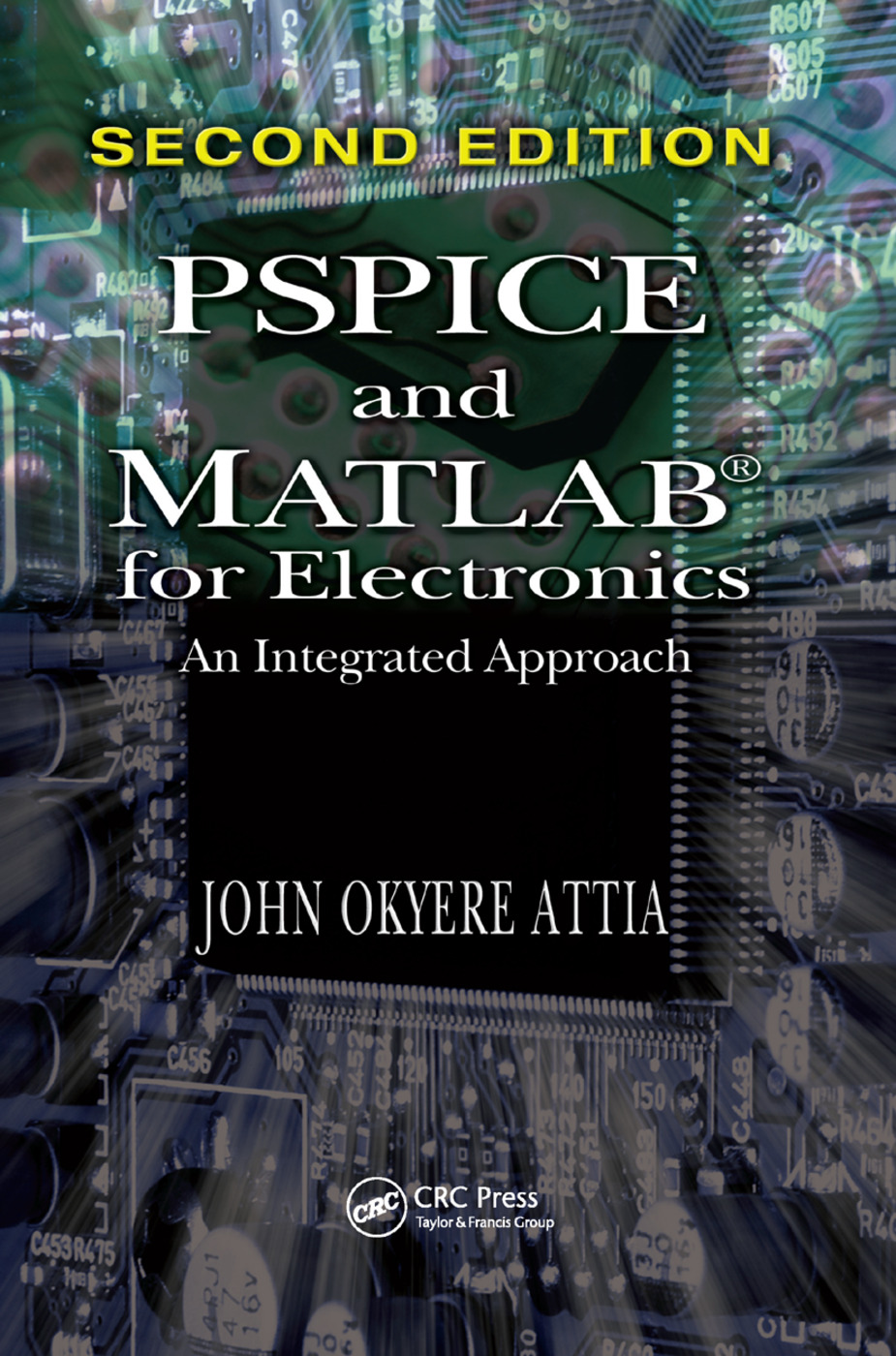PSPICE and MATLAB for Electronics

An Integrated Approach, Second Edition, 2nd Edition

CRC Press

382 pages | 233 B/W Illus.

New in Paperback: 9781138372740
pub: 2018-09-10
\$71.00
x
Hardback: 9781420086584
pub: 2010-06-23
\$105.00
x
eBook (VitalSource) : 9780429191930
pub: 2010-06-23
from \$35.50

FREE Standard Shipping!

Description

Used collectively, PSPICE and MATLAB® are unsurpassed for circuit modeling and data analysis. PSPICE can perform DC, AC, transient, Fourier, temperature, and Monte Carlo analysis of electronic circuits with device models and subsystem subcircuits. MATLAB can then carry out calculations of device parameters, curve fitting, numerical integration, numerical differentiation, statistical analysis, and two- and three-dimensional plots. PSPICE and MATLAB® for Electronics: An Integrated Approach, Second Edition illustrates how to use the strong features of PSPICE and the powerful functions of MATLAB for electronic circuit analysis.

After introducing the basic commands and advanced features of PSPICE as well as ORCAD schematics, the author discusses MATLAB fundamentals and functions. He then describes applications of PSPICE and MATLAB for problem solving. Applications covered include diodes, operational amplifiers, and transistor circuits.

New to the Second Edition

• Updated MATLAB topics
• Schematic capture and text-based PSPICE netlists in several chapters
• New chapter on PSPICE simulation using the ORCAD schematic capture program
• New examples and problems, along with a revised bibliography in each chapter

This second edition continues to provide anintroduction to PSPICE and a simple, hands-on overview of MATLAB. It also demonstrates the combined power of PSPICE and MATLAB for solving electronics problems. The book encourages readers to explore the characteristics of semiconductor devices using PSPICE and MATLAB and apply the two software packages for analyzing electronic circuits and systems.

Reviews

Praise for the First Edition

"… With the ease of using a high-level language, the book describes the combined power of PSPICE and MATLAB programming for analyzing diode, op-amps, and transistor circuits … These chapters are bound to motivate any enthusiastic electronic engineer interested in simulation, modeling, and analysis of electronic circuits. This book has clearly exposed the strength of the integrated usage of PSPICE and MATLAB packages in solving electronic circuits. The numerous examples, both worked out and homework problems, should provide the reader with a good knowledge of the integrated usage of PSPICE and MATLAB packages …"

—K. Vasudevan, University of Bridgeport, Connecticut, USA

"Each chapter contains a large number of worked PSPICE and MATLAB examples … Highly recommended as a useful addition for lower-division undergraduates through professionals, and two-year technical program students."

CHOICE, December 2002

Introduction

PSPICE Schematics

DC Analysis

PROBE

Transient Analysis

AC Analysis

PSPICE Fundamentals

Introduction

DC Analysis

Transient Analysis

AC Analysis

Printing and Plotting

Transfer Function Command

DC Sensitivity Analysis

Temperature Analysis

PROBE Statement

Device Model

Library File

Component Values (.PARAM, .STEP)

Function Definition (.FUNC, .INC)

Subcircuit (.SUBCKT, .ENDS)

Analog Behavioral Model

Monte Carlo Analysis (.MS)

Sensitivity and Worst Case Analysis (.WCASE)

Fourier Series (.FOUR)

MATLAB Fundamentals

MATLAB Basic Operations

Matrix Operations

Array Operations

Complex Numbers

The Colon Symbol

FOR Loops

IF Statements

Graph Functions

Input/Output Commands

MATLAB Functions

M-Files

Mathematical Functions

Data Analysis Functions

Derivative Function (diff)

Curve Fitting (polyfit, polyval)

Polynomial Functions (roots, poly, polyval, and fzero)

Interfacing SPICE to MATLAB

Diode Circuits

Diode

Rectification

Schematic Capture of Diode Circuits

Zener Diode Voltage Regulator

Peak Detector

Diode Limiters

Operational Amplifier

Inverting and Noninverting Configurations

Slew Rate and Full-Power Bandwidth

Schematic Capture of Operational Amplifier Circuits

Active Filter Circuits

Transistor Characteristics and Circuits

Characteristics of Bipolar Junction Transistors

MOSFET Characteristics

Biasing of BJT Circuits

MOSFET Bias Circuit

Frequency Response of Transistor Amplifiers

Schematic Capture of Transistor Circuits

Feedback Amplifiers

Each chapter contains a Bibliography at the end.

John Okyere Attia is a professor and head of the electrical and computer engineering department at Prairie View A&M University.